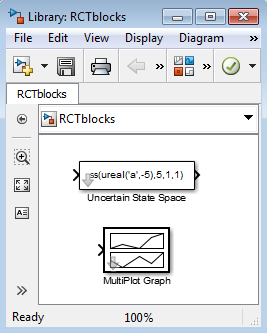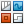Documentation

Analyzing Uncertainty in Simulink

Robust Control Toolbox™ software provides tools to model uncertain dynamics in Simulink®. Using these tools, you can analyze how the uncertainty impacts the time-domain and frequency-domain behavior of a Simulink model.

The Uncertain State Space block, included in the Robust Control Toolbox block library, is a convenient way to incorporate uncertainty information in a Simulink model. For more information, see Specify Uncertainty Using Uncertain State Space Blocks. Using this block, you can perform the following types of analysis:

• Vary the uncertainty and see how it affects the time responses (Monte Carlo analysis). See Simulate Uncertainty Effects.

• Analyze the effects of uncertainty on the linearized dynamics:

• If the operating point does not depend on the parameter uncertainty, use ulinearize to obtain an uncertain state-space model. You can then use usample to sample the uncertain variables and obtain a family of LTI models.

• If the operating point depends on the parameter uncertainty, use usample to sample the uncertainty and then use the Simulink Control Design™ linearize command to compute the linearized dynamics for each uncertainty value.

• Compute an uncertain linearization, i.e., obtain an uncertain state-space model (uss object) that combines the uncertain variables with the linearized dynamics. You can use this model to perform worst-case robustness analysis. See Obtain Uncertain State-Space Model from Simulink Model.

If you cannot use Uncertain State Space blocks in the Simulink model because you share the model or generate code, you can still compute an uncertain linearization by specifying a block to linearize to an uncertain variable. For example, you can specify a gain block to linearize to an uncertain real parameter (ureal). See Specify Uncertain Linearization for Core or Custom Simulink Blocks. You can then use the uncertain state-space model to analyze robustness in the linear operating range.

Simulink Blocks for Analyzing Uncertainty

Robust Control Toolbox software provides an Uncertain State Space block to model parametric and dynamic uncertainty in Simulink. The block library also contains a MultiPlot Graph block that you use with the Uncertain State Space block to plot and visualize Monte Carlo simulation responses.

To open the Robust Control Toolbox block library, type the following command at the MATLAB® prompt:

RCTblocks

The block library opens, as shown in the following figure.Alternatively, in a Simulink model window, clickto launch to Library Browser. In the Library Browser, select Robust Control Toolbox.